# Pizza palace

Josh is at Enzo's pizza palace. He can sit at a table with 5 of his friends or at a different table with seven of his friends. The same size pizza is shared equally among the people at each table. At which table should Josh sit to get more pizza? (write answer as number - 5 or 7)

x =  5

### Step-by-step explanation: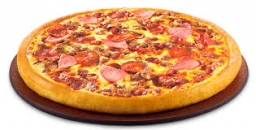Did you find an error or inaccuracy? Feel free to write us. Thank you!Tips to related online calculators
Need help to calculate sum, simplify or multiply fractions? Try our fraction calculator.

## Related math problems and questions:

• Equivalent expressionsA coach took his team out for pizza after their last game. There were 14 players, so they had to sit in smaller groups at different tables. Six players sat at one table and got 4 small pizzas to share equally. The other players sat at the different table
• Pizza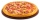Five friends were together for pizza. Adam divided his pizza into thirds, Boris in quarters, Denis in patina and Luke in sixth. Then Simon also came to them. Each of the five boys gave him one piece, leaving him one whole pizza. In how many equal parts di
• Pizza 5You have 2/4 of a pizza, and you want to share it equally between 2 people. How much pizza does each person get?
• Four people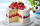Four people want waffles for breakfast. There are 6 waffles left. How can 6 waffles be shared equally among 4 people? How much does each person get? Draw a picture and write a division expression to model the problem.
• JerryJerry has 3/4 of a pizza. He needs to share it with 6 friends. What fraction of the pizza will each friend get? Only write the fraction
• Sort fractionsWhich is larger 3/7, 3/8, 3/9, 3/6 =
• Three friendsThree friends had balls in ratio 2: 7: 4 at the start of the game. Could they have the same number of balls at the end of the game? Write 0, if not, or write the minimum number of balls they had together.
• Barbara 2Barbara get 6 pizzas to divide equally among 4 people. How much of a pizza can each person have?
• Pizza fractionsAnn ate a third of a pizza and then another quater. Total part of pizza eaten by Ann and how much pizza is left?
• Christmas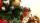Exactly after 114 hours, we sit down at the Christmas Eve table. What day and what time it was when Dad said this sentence. At the Christmas Eve table, they sit down exactly at the 18-o'clock (6 PM).
• Divide 11Divide the product of 4 and 5/8 by 1 1/2. Write your answer as a mixed number.
• Table and chairs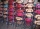Four people should sit at a table in front of a row of 7 chairs. What is the probability that there will be no empty chair between them if people choose their place completely at random?
• Pizza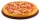Three siblings ordered one pizza. Miška ate a quarter of the whole pizza. Lenka ate a third of the rest and Patrik ate half of what Lenka had left. They had the rest packed up. How much of the pizza did they pack? Write the result as a fraction.
• Division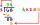Divide by the number 0.2 is the same as multiply by what number?
• Table 3x3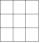Table with numbers 3x3 in the first row 66,24,33 in the second row 57,? 19 in the third row 18,45,60 What number comes ar mark ?. Maybe the numbers: 22,46,45,47
• FractionsSort fractions z1 = (6)/(11); z2 = (10)/(21); z3 = (19)/(22) by its size. Result write as three serial numbers 1,2,3.
• Two friends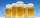Two friends met as a good man perish together for a beer. After recovery the most important topics (politics, women, football ...), one asks: - And how many do you have children? - I have 3 children. - And how many years have? Friend already not want to a